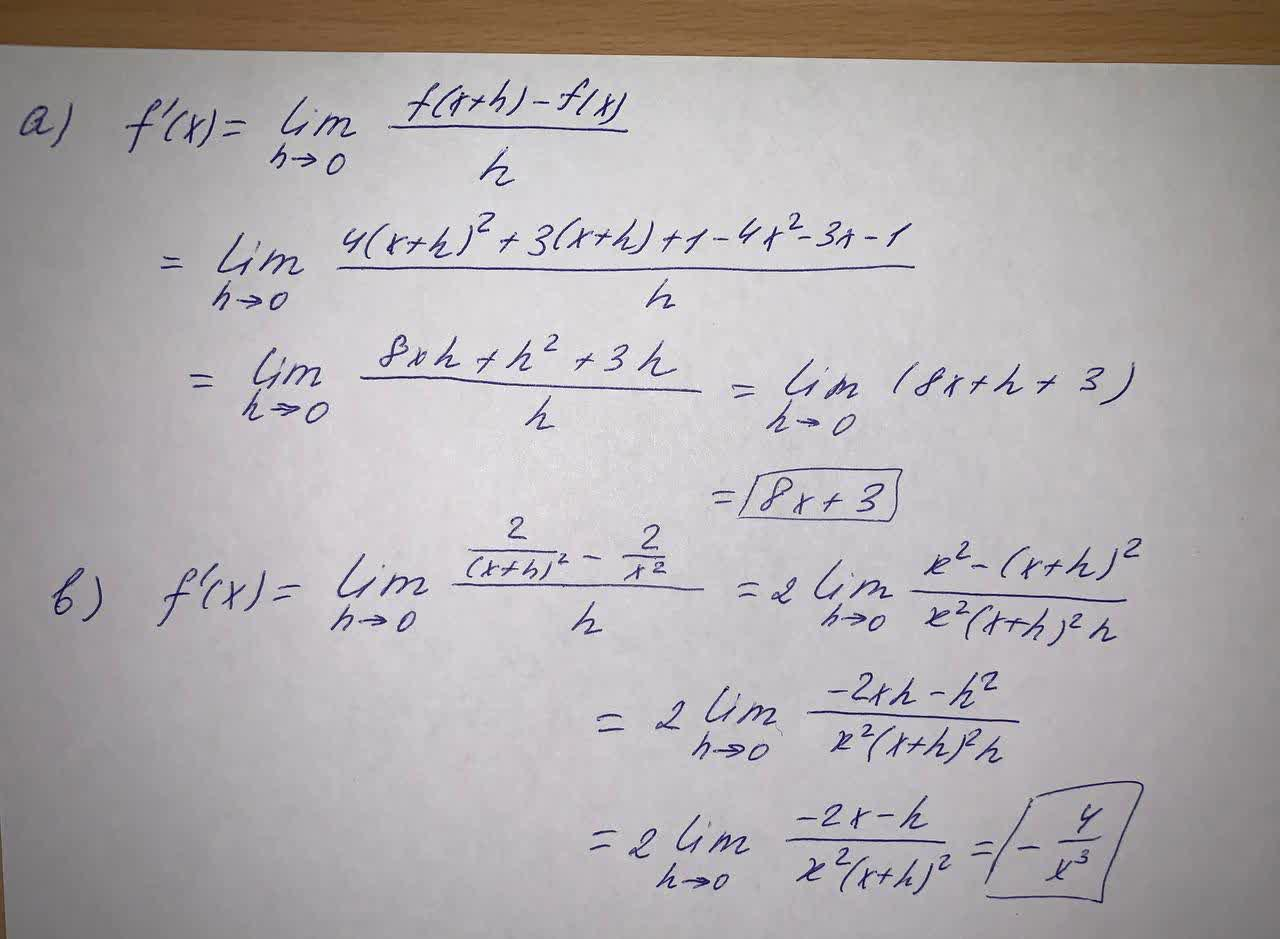Question# Use the limit definition of the derivative to calculate the derivatives of the following function: (a) f(x)=4x^2+3x+1 (b) f(x)=\frac{2}{x^2}

Limits and continuity
ANSWEREDUse the limit definition of the derivative to calculate the derivatives of the following function:
(a) $$f(x)=4x^2+3x+1$$
(b) $$f(x)=\frac{2}{x^2}$$